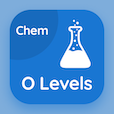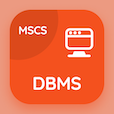Cambridge Online Courses (GCSE)

O Level Chemistry MCQs

O Level Chemistry MCQ PDF - Topics

# Chemical formulas MCQ Quiz Online

Learn Chemical formulas Multiple Choice Questions (MCQ), Chemical formulas quiz answers PDF to study o level chemistry online course for o level chemistry classes. Chemical Formulae and Equations Multiple Choice Questions and Answers (MCQs), Chemical formulas quiz questions for ACT subject tests. "Chemical formulas MCQ" PDF Book: valency and chemical formula, chemical symbols, chemical formulas test prep for GRE subject tests.

"The chemical formula of silver bromide is" MCQ PDF: chemical formulas with choices ag2br3, agbr, agbro, and agbr3.h2o for ACT subject tests. Study chemical formulas quiz questions for merit scholarship test and certificate programs for online bachelor degree programs.

## MCQs on Chemical formulas Quiz

MCQ: The chemical formula of silver bromide is

Ag2Br3
AgBr
AgBrO
AgBr3.H2O

MCQ: The chemical formula of Caustic soda is

NaOH
KOH
MgO
Ca(OH)2

MCQ: The chemical formula of monohydrate monosodium phosphate is

H2ONaPO2
PO2NaH2O
NaH2PO4.H2O
NaH3PO2.H2O

MCQ: The chemical formula of calcium nitrate is

CNOH
Ca(NO3)2
CaNO3
CaNO3

MCQ: The chemical formula of rouge pigment is

Fe2O3
Fe3O4
FeSO4
FeNO3

### More Topics from O Level Chemistry Course